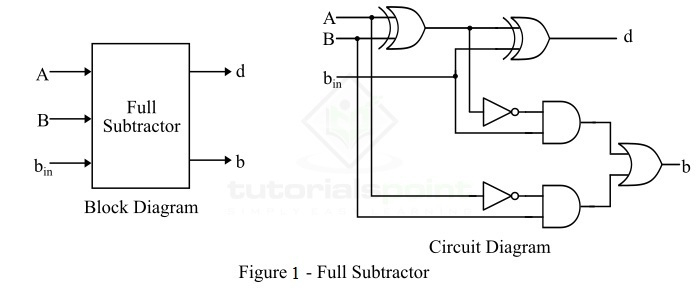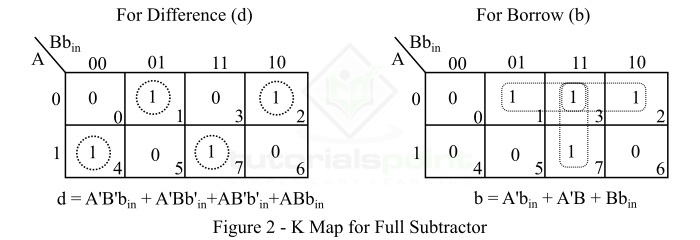# Full Subtractor in Digital Electronics

In digital electronics, a subtractor is a combinational logic circuit that can perform the subtraction of two number (binary numbers) and produce the difference between them. It is a combinational circuit that means its output depends on its present inputs only. Although, in practice, the subtraction of two binary number is accomplished by taking the 1's or 2's compliment of the subtrahend and adding it to the minuend. In this way, the subtraction operation of binary numbers can be converted into simple addition operation which makes hardware construction simple and less expensive. There are two types of subtractors namely, Half Subtractor and Full Subtractor.

In this article, we will discuss the full subtractor, its basic definition, circuit diagram, truth table, characteristic equation, k-map, etc. So let's begin with the basic definition of full subtractor.

## What is a Full-Subtractor?

A full-subtractor is a combinational circuit that has three inputs A, B, bin and two outputs d and b. Where, A is the minuend, B is subtrahend, bin is borrow produced by the previous stage, d is the difference output and b is the borrow output.

As we know that the half-subtractor can only be used for subtraction of LSB (least significant bit) of binary numbers. If there is any borrow during the subtraction of the LSBs of two binary numbers, then it will affect the subtraction of next stages. Therefore, the subtraction with borrow are performed by a full subtractor.

The block diagram and circuit diagram of a full-subtractor is shown in Figure-1.Therefore, we can realize the full-subtractor using two XOR gates, two NOT gates, two AND gates, and one OR gate.

## Operation of Full Subtractor

Now, let us understand the operation of the full subtractor. Full subtractor performs its operation to find the difference of two binary numbers according to the rules of binary subtraction, which are as follows −

In the case of full subtractor, the 1s and 0s for the output variables (difference and borrow) are determined from the subtraction of A – B – bin.

From the logic circuit diagram of the full subtractor, it is clear that the difference bit (d) is obtained by the XOR operation of the two inputs A, B, and bin, and the output borrow bit (b) is obtained by NOT, AND, and OR operations of variable A, B, and bin.

## Truth Table of Full-Subtractor

The truth table is one that gives relationship between input and output of a logic circuit. The following is the truth table of the full-subtractor −

Inputs Outputs
A B bin d (Difference) b (Borrow)
0 0 0 0 0
0 0 1 1 1
0 1 0 1 1
0 1 1 0 1
1 0 0 1 0
1 0 1 0 0
1 1 0 0 0
1 1 1 1 1

## K-Map for Full Subtractor

We can use the K-Map (or Karnaugh Map), a method for simplifying Boolean algebra, to determine equations of the difference bit (d) and the output borrow bit (b).

The K-Map simplification for half subtractor is shown in Figure-2.## Characteristic Equations of Full Subtractor

The characteristic equations of the full subtractor, i.e. equations of the difference (d) and borrow output (b) are obtained by following the rules of binary subtraction. These equations are given below −

The difference (d) of the full subtractor is the XOR of A, B, and bin. Therefore,

$$\mathrm{Difference,\, d=A\oplus B\oplus b_{in}=A'B'b_{in}+AB'b'_{in}+A'Bb'_{in}+ABb_{in}}$$

The borrow (b) of the full subtractor is given by,

From the logic circuit diagram and k-map −

$$\mathrm{Borrow,\, b=A'B+\left ( A\oplus B \right )'b_{in}}$$

From truth table

$$\mathrm{Borrow,\, b=A'B'b_{in}+A'Bb'_{in}+A'Bb_{in}+ABb_{in}}$$

Or

$$\mathrm{Borrow,\, b=A'B\left ( b_{in}+b'_{in} \right )+\left (AB+A'B' \right )b_{in}=A'B+\left (A\oplus B \right )'b_{in}}$$

## Applications of Full Subtractor

The following are some important applications of full subtractor −

• Full subtractors are used in ALU (Arithmetic Logic Unit) in computers CPUs.

• Full subtractors are extensively used to perform arithmetical operations like subtraction in electronic calculators and many other digital devices.

• Full subtractors are used in different microcontrollers for arithmetic subtraction.

• They are used in timers and program counters (PC).

• Full subtractors are also used in processors to compute addresses, tables, etc.

• Full subtractors are also used in DSP (Digital Signal Processing) and networking based systems.

## Conclusion

From the above discussion, we can conclude that a full-subtractor is a combinational logic circuit that can compute the difference of three binary digits. In a full subtractor, the borrow (if any) from the previous stage is also used in subtraction operation in the next stages. Therefore, full subtractors are used to perform subtraction of binary numbers having any number of digits.